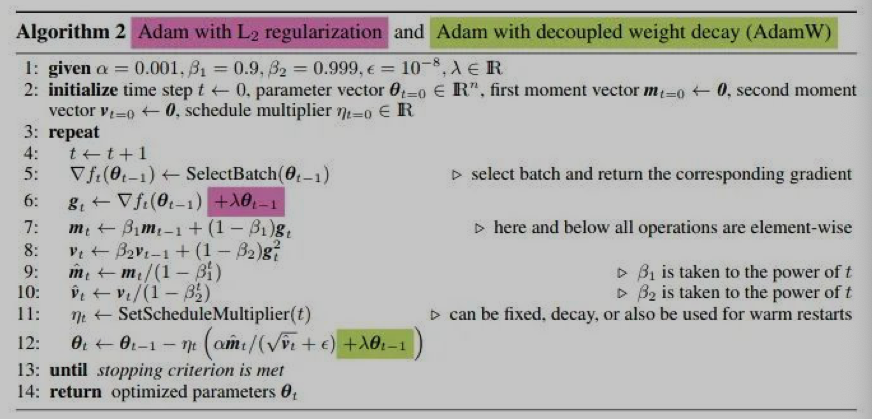# 从 SGD 到 AdamW 原理和代码解读

1. 计算目标函数关于当前参数的梯度: $g_t = ∇f(w_t)$
2. 根据历史梯度计算一阶动量和二阶动量: $m_t = \phi(g_1,g_2,…,g_t); V_t =\psi(g_1,g_2,…,g_t)$
3. 计算当前时刻的下降梯度: $\eta = \alpha \cdot m_t/\sqrt V_t$
4. 根据下降梯度进行更新: $w_{t+1} = w_t -\eta_t$

• add_param_group(param_group) : 把参数放进优化器中，这在Fine-tune预训练时可以使冻结层可训练，并随着训练的进行添加到优化器中。
• state_dict():返回优化器状态，以dict形式
• step(closure=None):优化一步参数

## SGD

SGD没有动量的概念，也就是说：

SGD最大的缺点就是下降速度慢，而且可能会在沟壑的两边持续震荡，停留在一个局部最优点。

## SGD with Momentum

$\beta_1$的经验值为0.9，这意味着下降方向主要是此前累积的下降方向，并略微偏向当前时刻的下降方向。想象高速公路上汽车转弯，在高速向前的同时略微偏向，急转弯可是要出事的。

## SGD with Nesterov Acceleration

SGD还有一个问题是困在局部最优的沟壑里震荡。想象一下你走到一个盆地，四周都是略高的小山，你觉得没有下坡的方向，那就只能呆在这里了。可是你如果爬上高地，就会方向外卖的世界还很广阔。

• params (iterable) – 优化器作用的模型参数。
• lr (float) – learning rate，相当于是统一框架中的 $\alpha$
• momentum (float, optional) – 动量参数。(默认值：0)
• weight_decay (float, optional) – 权重衰减系数 weight decay (L2 penalty) (默认值：0)
• dampening (float, optional) – dampening for momentum (默认值：0)
• nesterov (bool, optional) – 允许 Nesterov momentum (默认值：False)

SGD及其变种以同样的学习率更新每个参数，但深度神经网络往往包含大量的参数，这些参数并不是总会用得到(想想大规模的embedding)。

• params (iterable) – 优化器作用的模型参数。
• lr (float) – learning rate – 相当于是统一框架中的 。
• lr_decay(float,optional) – 学习率衰减 (默认值：0)
• weight_decay (float, optional) – 权重衰减系数 weight decay (L2 penalty) (默认值：0)
• eps(float,optional)：防止分母为0的一个小数 (默认值：1e-10)

## RMSProp

• params (iterable) – 优化器作用的模型参数。
• lr (float) – learning rate – 相当于是统一框架中的 $\alpha$。
• momentum (float, optional) – 动量参数。(默认值：0)。
• alpha(float,optional) – 平滑常数 (默认值：0.99)。
• centered(bool,optional) – ifTrue, compute the centered RMSProp, the gradient is normalized by an estimation of its variance，就是这一项是 True 的话就把方差使用梯度作归一化。
• weight_decay (float, optional) – 权重衰减系数 weight decay (L2 penalty) (默认值：0)
• eps(float,optional)：防止分母为0的一个小数 (默认值：1e-10)

SGD的一阶动量：

• params (iterable) – 优化器作用的模型参数。
• lr (float) – learning rate – 相当于是统一框架中的 。
• betas(Tuple[float,float],optional) – coefficients used for computing running averages of gradient and its square ((默认值：(0.9, 0.999))
• weight_decay (float, optional) – 权重衰减系数 weight decay (L2 penalty) (默认值：0)
• eps(float,optional)：防止分母为0的一个小数 (默认值：1e-10)Just adding the square of the weights to the loss function is not the correct way of using L2 regularization/weight decay with Adam, since that will interact with the m and v parameters in strange ways. Instead we want to decay the weights in a manner that doesn’t interact with the m/v parameters. This is equivalent to adding the square of the weights to the loss with plain (non-momentum) SGD. Add weight decay at the end (fixed version).

• params (iterable) – 优化器作用的模型参数。
• lr (float) – learning rate – 相当于是统一框架中的 。
• betas(Tuple[float,float],optional) – coefficients used for computing running averages of gradient and its square ((默认值：(0.9, 0.999))
• weight_decay (float, optional) – 权重衰减系数 weight decay (L2 penalty) (默认值：0)
• eps(float,optional)：防止分母为0的一个小数 (默认值：1e-10)

Adam如果使用weight_decay的话，那么相当于目标函数上加了$1/2\gamma||\theta||^2$，所以相当于是梯度加上$\gamma\theta$故Adam使用了

$\thetat =\theta{t-1}-\alpha\cdot\lambda\cdot\theta_{t-1}-\alpha\cdot\eta_t$